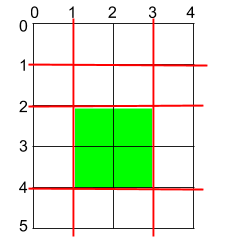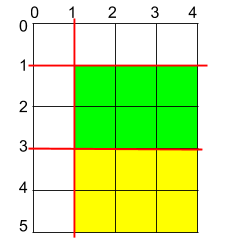@TOC

# # 题目描述``````输入：h = 5, w = 4, horizontalCuts = [1,2,4], verticalCuts = [1,3]

````````````输入：h = 5, w = 4, horizontalCuts = [3,1], verticalCuts = 

``````

``````输入：h = 5, w = 4, horizontalCuts = , verticalCuts = 

``````

1. `2 <= h, w <= 10^9`
2. `1 <= horizontalCuts.length < min(h, 10^5)`
3. `1 <= verticalCuts.length < min(w, 10^5)`
4. `1 <= horizontalCuts[i] < h`
5. `1 <= verticalCuts[i] < w`
6. 题目数据保证 `horizontalCuts` 中的所有元素各不相同
7. 题目数据保证 `verticalCuts` 中的所有元素各不相同

# # 解题方法

## # 找最大间隔之积

1. `1 <= horizontalCuts[i] <= max(horizontalCuts)`
2. `1 <= verticalCuts[j] <= max(verticalCuts)`

Python 代码如下：

``````class Solution:
def maxArea(self, h: int, w: int, horizontalCuts: List[int], verticalCuts: List[int]) -> int:
horizontalCuts.append(0); horizontalCuts.append(h)
verticalCuts.append(0); verticalCuts.append(w)
horizontalCuts.sort()
verticalCuts.sort()
M, N = len(horizontalCuts), len(verticalCuts)
max_hc = 0
max_vc = 0
for i in range(M - 1):
max_hc = max(max_hc, horizontalCuts[i + 1] - horizontalCuts[i])
for j in range(N - 1):
max_vc = max(max_vc, verticalCuts[j + 1] - verticalCuts[j])
return (max_hc * max_vc) % (10 ** 9 + 7)
``````

# # 日期

2020 年 6 月 1 日 —— 6月的开始，儿童节快乐！